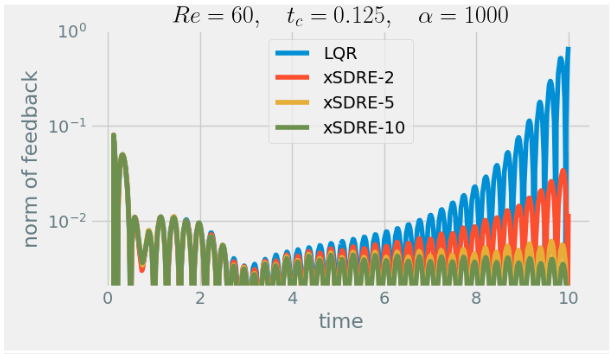# Low-complexity LPV approximations of incompressible Navier-Stokes equations for truncated state-dependent Riccati feedback### Abstract

Nonlinear feedback design via state-dependent Riccati equations is well established but unfeasible for large-scale systems because of computational costs. If the system can be embedded in the class of linear parameter-varying (LPV) systems with the parameter dependency being affine-linear, then the nonlinear feedback law has a series expansion with constant and precomputable coefficients. In this work, we propose a general method to approximating nonlinear systems such that the series expansion is possible and efficient even for high-dimensional systems. We lay out the stabilization of incompressible Navier- Stokes equations as application, discuss the numerical solution of the involved matrix-valued equations, and confirm the performance of the approach in a numerical example.

Type